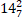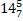# Quiz Discussion

The average weight of 3 men, A, B and C is 84 kg. Another man D joins the group and the average now becomes 80 kg. If another man E whose weight is 3 kg more than that of D, replaces A then the average weight of B, C, D and E becomes 79 kg. What is the weight of A ?

Course Name: Quantitative Aptitude

• 1] 70 kg
• 2] 72 kg
• 3] 75 kg
• 4] 80 kg
##### Solution
No Solution Present Yet

#### Top 5 Similar Quiz - Based On AI&ML

Quiz Recommendation System API Link - https://fresherbell-quiz-api.herokuapp.com/fresherbell_quiz_api

# Quiz
1
Discuss

The average age of 30 students is 9 years. If the age of their teacher is included, the average age becomes 10 years. The age of the teacher (in years) is :

• 1] 27
• 2] 31
• 3] 35
• 4] 40
##### Solution
2
Discuss

The average income of A, B and C is Rs. 12,000 per month and average income of B, C and D is Rs. 15,000 per month. If the average salary of D be twice that of A, then the average salary of B and C is in Rs. :

• 1] 8,000
• 2] 18,000
• 3] 13,500
• 4] 9,000
##### Solution
3
Discuss

The average age of a family of 10 members is 20 years. If the age of the youngest member of the family is 10 years, then the average age of the members of the family just before the birth of the youngest member was approximately.

• 1]

27.14 years

• 2]

12.5 years

• 3]

14.28 years

• 4]

$$11\frac{1}{9}$$ years

##### Solution
4
Discuss

A school has only four classes that contain 10, 20, 30 and 40 students respectively. The pass percentage of these classes are 20%, 30%, 60% and 100% respectively. Find the pass % of the entire school.

• 1] 56%
• 2] 76%
• 3] 34%
• 4] 66%
• 5] None of these
##### Solution
5
Discuss

The average of 20 numbers is zero. Of them, at the most, how many may be greater than zero ?

• 1]

0

• 2]

-1

• 3]

1

• 4]

None Of These

##### Solution
6
Discuss

The average of 6 observations is 45.5. If one new observation is added to the previous observations, then the new average becomes 47. The new observation is :

• 1] 58
• 2] 56
• 3] 50
• 4] 46
##### Solution
7
Discuss

Find the average of the first 40 natural numbers.

• 1]

21

• 2]

20.5

• 3]

21.5

• 4]

None Of These

##### Solution
8
Discuss

The average of runs scored by a cricketer in his 99 innings is 99. How many runs will he have to score to his 100th innings so that his average of runs in 100 innings will become 100 ?

• 1] 100
• 2] 99
• 3] 199
• 4] 101
##### Solution
9
Discuss

A man started his journey from Lucknow to Kolkata, which is 200 km, at the speed of 40 kmph then he went to Banglore which is 300 km, at the speed of 20 kmph. Further he went to Ahmedabad which is 500 km, at the speed of 10 kmph. The average speed of the man is :

• 1]kmph

• 2]Kmph

• 3]

15.6 kmph

• 4]

16.1 kmph

##### Solution
10
Discuss

The average of five numbers is 306.4. The average of the first two numbers is 431 and the average of the last two numbers is 214.5. What is the third number?

• 1] 52
• 2] 108
• 3] 321
• 4] Cannot be determined
• 5] None of these
# Quiz